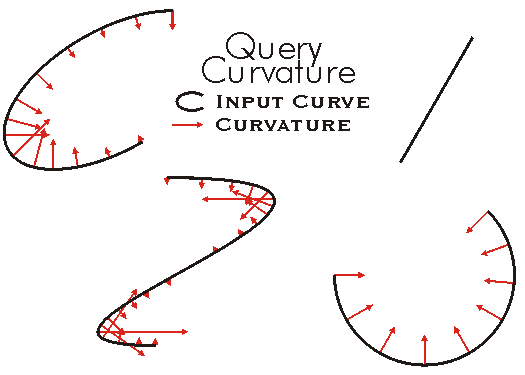This document is archived and information here might be outdated.  Recommended version.

ISegment.QueryCurvature Method (ArcObjects .NET 10.8 SDK)
 ArcObjects Help for .NET developers > ArcObjects Help for .NET developers > ArcObjects namespaces > Geometry > ESRI.ArcGIS.Geometry > Interfaces > IS > ISegment Interface > ISegment.QueryCurvature Method
 ArcGIS Developer Help

# ISegment.QueryCurvature Method

Finds curvature and unit vector starting at point on segment and directed to embedded circle center.

```[Visual Basic .NET]
Public Sub QueryCurvature ( _
ByVal DistanceAlongCurve As Double, _
ByVal asRatio As Boolean, _
ByRef curvature As Double, _
ByVal unitVector As ILine _
)
```
```[C#]
public void QueryCurvature (
double DistanceAlongCurve,
bool asRatio,
ref double curvature,
ref ILine unitVector
);
```
```[C++]
HRESULT QueryCurvature(
double DistanceAlongCurve,
VARIANT_BOOL asRatio,
System.Double* curvature,
ILine* unitVector
);
```
```[C++]
Parameters
DistanceAlongCurve
DistanceAlongCurve is a parameter of type double
asRatio
asRatio is a parameter of type bool
curvature [out]
curvature is a parameter of type double*
unitVector
unitVector is a parameter of type ILine*
```

#### Product Availability

Available with ArcGIS Engine, ArcGIS Desktop, and ArcGIS Server.

#### Description

Returns the curvature at a specified distance along the segment.  The distance can be given explicitly or as a ratio of the entire length of the segment.  The curvature is returned as a Line representing a directional unit vector (in the direction going from the From Point to the To Point) and a double representing the magnitude of the vector of curvature.

#### Remarks

The unit vector returned by QueryCurvature points toward the center of the circle that has the same first and second derivative at the queried point with a curvature value equal to 1 / Radius of that circle.#### See Also

ISegment Interface